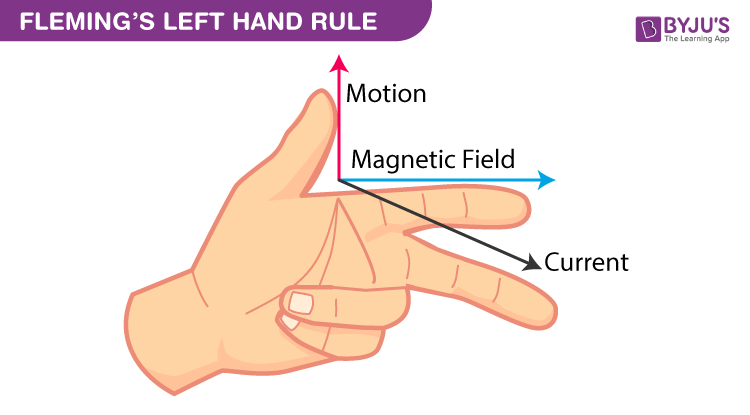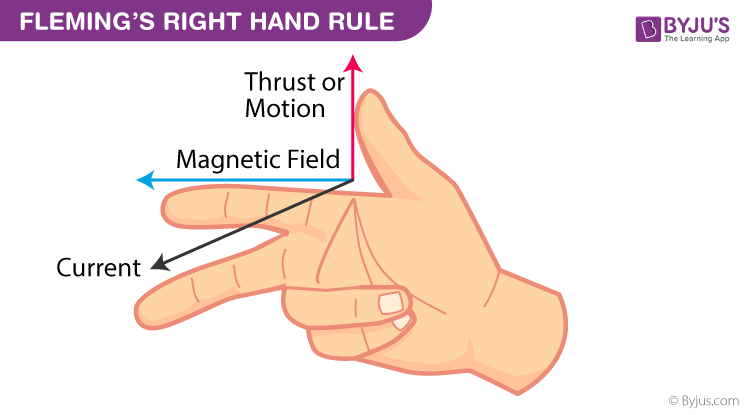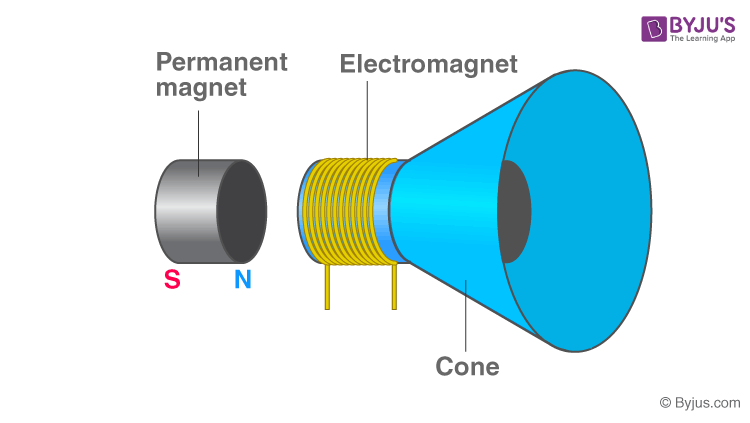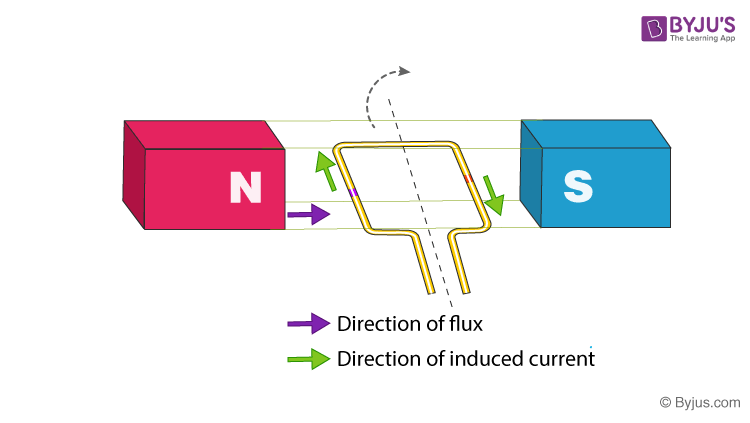# Electromagnetism

Electromagnetism is a branch of Physics, that deals with the electromagnetic force that occurs between electrically charged particles. The electromagnetic force is one of the four fundamental forces and exhibits electromagnetic fields such as magnetic fields, electric fields, and light. It is the basic reason electrons are bound to the nucleus and are responsible for the complete structure of the nucleus.

## What is Electromagnetic Force?

The electromagnetic force is a type of physical interaction that occurs between electrically charged particles. It acts between charged particles and is the combination of all magnetic and electrical forces. The electromagnetic force can be attractive or repulsive.

Before the invention of electromagnetism, people or scientists used to think electricity and magnetism are two different topics. The view has changed after James Clerk Maxwell published A Treatise on Electricity and Magnetism in the year 1873. The publication states that the interaction of positive and negative charges are mediated by one force. This observation laid a foundation for Electromagnetism. Later many scientists like Michael Faraday, Oliver Heaviside, and Heinrich Hertz contributed their ideas in electromagnetism.

Watch the video below to understand the concept of electromagnetism in detail.## What is Electromagnetism?

Electromagnetism is a process where a magnetic field is created by introducing the current in the conductor. When a conductor is electrically charged it generates magnetic lines of force of conductor. For example, if current i.e., positive charges moving in a wire, it produces the magnetic field along the wire, and the direction of magnetic lines and force can be determined using Right-hand Rule. Refer to the image for a detailed explanation.## Explanation of Electromagnetism with an Example

Permanent Magnetic speakers commonly used in TV’s and Radios are perfect examples of Electromagnetic devices. Let’s see the operation of these devices which are based on the principle of electromagnetism. See the picture below.In order to convert electrical waves into audible sound, the speakers are designed. A metal coil is attached to a permanent magnet and when current passes through the coil it generates a magnetic field. The newly formed magnetic field is repelled by the permanent magnetic field resulting in the vibrations. These vibrations are amplified by the cone-like structure causing the sound. This is how speakers work based on electromagnetism.

### Electromagnetic Induction

We have seen what happens when a conductor is electrically charged. Now, let’s see what happens if we place a conductor in between the magnetic field.

When a conductor is placed or moved through the magnetic field it generates voltage i.e., electricity. This principle is called Electromagnetic Induction. The voltages generated will be based on the speed of the conductor moving through the electric field. Faster the speed of the conductor, the greater the induced electricity or voltage.

According to  Faraday’s Law, the relative motion between magnetic field and conductor, the flux linkage changes and this change in flux induces a voltage across the coil.

### Explanation with an example

DC Generator works on the principle of Faraday’s Law of Electromagnetic Induction. It is a system that converts mechanical energy into electrical energy.In the above figure, A rectangular conductor width sides are placed in between a magnetic field. When the rectangular conductor rotates in between magnetics, it cuts the magnetic field thereby causing the Electromagnetic field (e m f).

## Properties of the Electromagnetic Wave

A few properties of electromagnetic waves are:

• Electromagnetic waves are propagated by oscillating electric and magnetic waves at right angles to each other.
• They exhibit the properties of interference and diffraction.
• They travel at a speed of 3 × 108 m/s in a vacuum.
• They are transverse waves.
• The relationship between the wavelength (λ) and frequency (c) of an electromagnetic wave is given as follows:

c = v λ

## Applications of Electromagnetism

A few applications of electromagnetism are:

• Electromagnetism serves as a fundamental working principle for many of the home appliances in household applications.
• The Maglev trains or high-speed trains work on the principle of electromagnetism.
• Electromagnetic radiations are used in the communication system to transfer data from the source to the receiver.
• In industries, starting from small instruments to large power equipment, electromagnetism is used at least at one stage of their work.

## Frequently Asked Questions – FAQs

### What is Electromagnetism?

Electromagnetism is a branch of physics that involves the study of electromagnetic force. It is a type of interaction that occurs between electrically charged particles.

### Define electromagnetic force?

The electromagnetic force is a force that acts between charged particles and is a combination of electrical and magnetic forces.

Faraday’s Law states that whenever there is relative motion between magnetic field and conductor, the flux linkage changes and this change in flux induces a voltage across the coil.

### Define electromagnetic induction?

Electromagnetic induction is the production of an electromotive force across an electrical conductor in a changing magnetic field.

### How are electromagnetic waves propagated?

Electromagnetic waves are propagated by oscillating electric and magnetic waves at right angles to each other.

1. Filemon

This is useful, I learned more from it. Thank you very much.

2. nuwahereza brian

best definitions

3. IMANIRAGUHA SAMUEL

Perfect

4. Simion Jomo

I was able to grasp the content so fast. Thanks for such a helpful work.